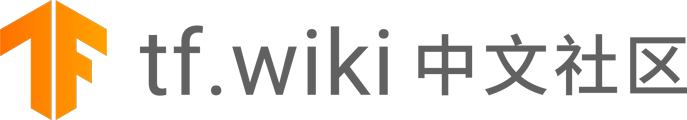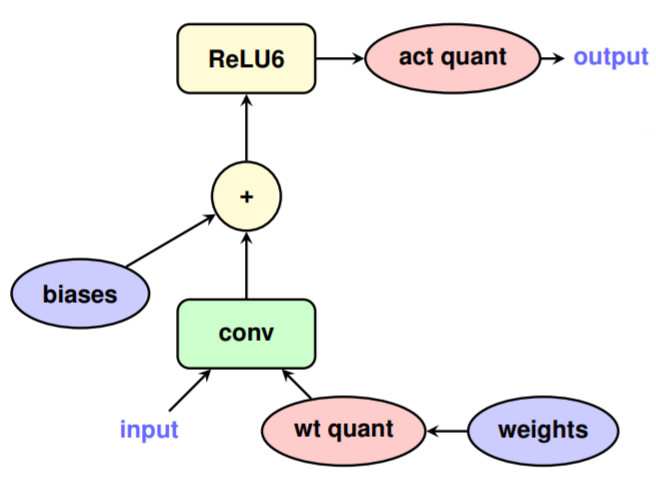# TensorFlow 模型优化工具包 — 训练时量化

## 训练时量化

QAT 的核心思想是，可以在训练过程的正向传递中模拟低精度的推理时间计算。这项 研究成果 要归功于 Google 移动视觉团队成员 Skirmantas Kligys 的原始创新。它将量化误差作为训练过程中的干扰并计入整体损失，而优化算法会尽量将它降至最低。因此，该模型可学习对量化更稳健的参数。

## QAT 训练的模型具有与浮点相当的准确率

### 执行量化模拟操作“‘wt quant”和“act quant”算子在模型的正向传递中引入了损失，以便模拟推理过程中的实际量化损失。请注意，Conv 和 ReLU6 之间没有量化操作。这是因为 ReLU 已融入 TensorFlow Lite

## 通过几行代码使用此 API

QAT API 为量化 TensorFlow Keras 模型提供了一种简单且高度灵活的方法。借此，您可以非常轻松地使用 “训练时量化” 训练整个或部分模型，然后将其导出，以便通过 TensorFlow Lite 进行部署。

### 量化整个 Keras 模型

``````import tensorflow_model_optimization as tfmot

model = tf.keras.Sequential([
...
])
# Quantize the entire model.
quantized_model = tfmot.quantization.keras.quantize_model(model)

# Continue with training as usual.
quantized_model.compile(...)
quantized_model.fit(...)
``````

### 量化部分 Keras 模型

``````import tensorflow_model_optimization as tfmot
quantize_annotate_layer = tfmot.quantization.keras.quantize_annotate_layer

model = tf.keras.Sequential([
...
# Only annotated layers will be quantized.
quantize_annotate_layer(Conv2D()),
quantize_annotate_layer(ReLU()),
Dense(),
...
])

# Quantize the model.
quantized_model = tfmot.quantization.keras.quantize_apply(model)
``````

## 致谢